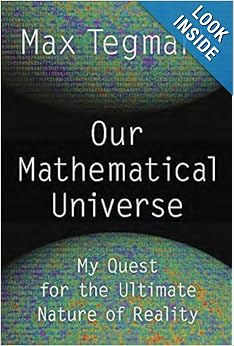# Max Tegmark’s “Our Mathematical Universe”Physicist Max Tegmark has just published an interesting new book entitled Our Mathematical Universe: My Quest for the Ultimate Nature of Reality. In this very lucidly written book, Tegmark takes the reader on a tour of modern physics and then introduces his theory of the ultimate nature of the universe.

Tegmark starts out by exploring a list of questions, such as “How big is space?,” “Where did our solar system come from?” and “Where did our big bang come from?.” He then examines some of the difficulties with current theories, including both the successes and failures of the “inflation” cosmology of the very early universe.

Next, Tegmark introduces the emerging “multiverse” theories: Level I (the universe external to ours, as a result of the inflation at the big bang), Level II (the ensemble of pocket universes, the result of “eternal inflation”), Level III (the bifurcating universe, as a consequence of the “many worlds” formulation of quantum mechanics) and Level IV (Tegmark’s own notion of all mathematical structures, which he proposes actually exist and constitute the ultimate “stuff” of existence).

Of particular interest to readers of this column is the last portion of the book, chapters 10-13. Tegmark starts out by noting that scientists for centuries have noted how the universe is governed by mathematical laws. Galileo wrote that the book of the universe “is written in the language of mathematics.” Wigner, writing in 1960, remarked that “the enormous usefulness of mathematics in the natural sciences is something bordering on the mysterious and … there is no rational expiation for it.”

Tegmark then proposes his own theory to explain Wigner’s paradox. He starts by proposing two hypotheses:

1. (External reality hypothesis): There exists an external physical reality completely independent of us humans.
2. (Mathematical universe hypothesis): Our external physical reality is a mathematical structure.

He points out that if reality exists independently of humans, then a complete description must be well-defined according to nonhuman entities (aliens or supercomputers) that lack human concepts. He then points out that a completely human-baggage-free description is precisely a mathematical structure: by the mathematical definition of equivalence, two descriptions are equivalent if there is a correspondence between them that preserves all relations. But a set of abstract entities with relations between them is a mathematical structure.

With this introduction, Tegmark proposes his Level IV multiverse: the set of all mathematical structures, all of which actually exist, although the vast majority of them contain no sentient beings to wonder about the nature of their existence.

Tegmark points out that the mathematical universe hypothesis (MUH) solves the “infinite regress” problem, in which nature can only be explained from its parts, which requires a deeper explanation, etc. This is because the ultimate building blocks have no properties at all, only relations between them. The MUH also solves Stephen Hawking’s quip “what breathes fire into the equations?” There is no need of anyone breathing fire, because the universe is mathematics.

Before closing, Tegmark proposes one additional hypothesis: the computable universe hypothesis, namely that the mathematical structure that is the external physical reality is defined by computable functions, namely that all computations involved are guaranteed to halt after a finite number of steps.

Although some might be dismayed at the thought that we are nothing more than mathematical structures, insignificant in a vast cosmos, Tegmark notes that the future of our planet, and perhaps of life in the Milky Way or beyond, may be decided in our lifetime. So let’s make a difference!

For additional details and discussion, see a Huffington Post report, a UK Guardian review, a Wall Street Journal review, and a New York Journal of Books review.